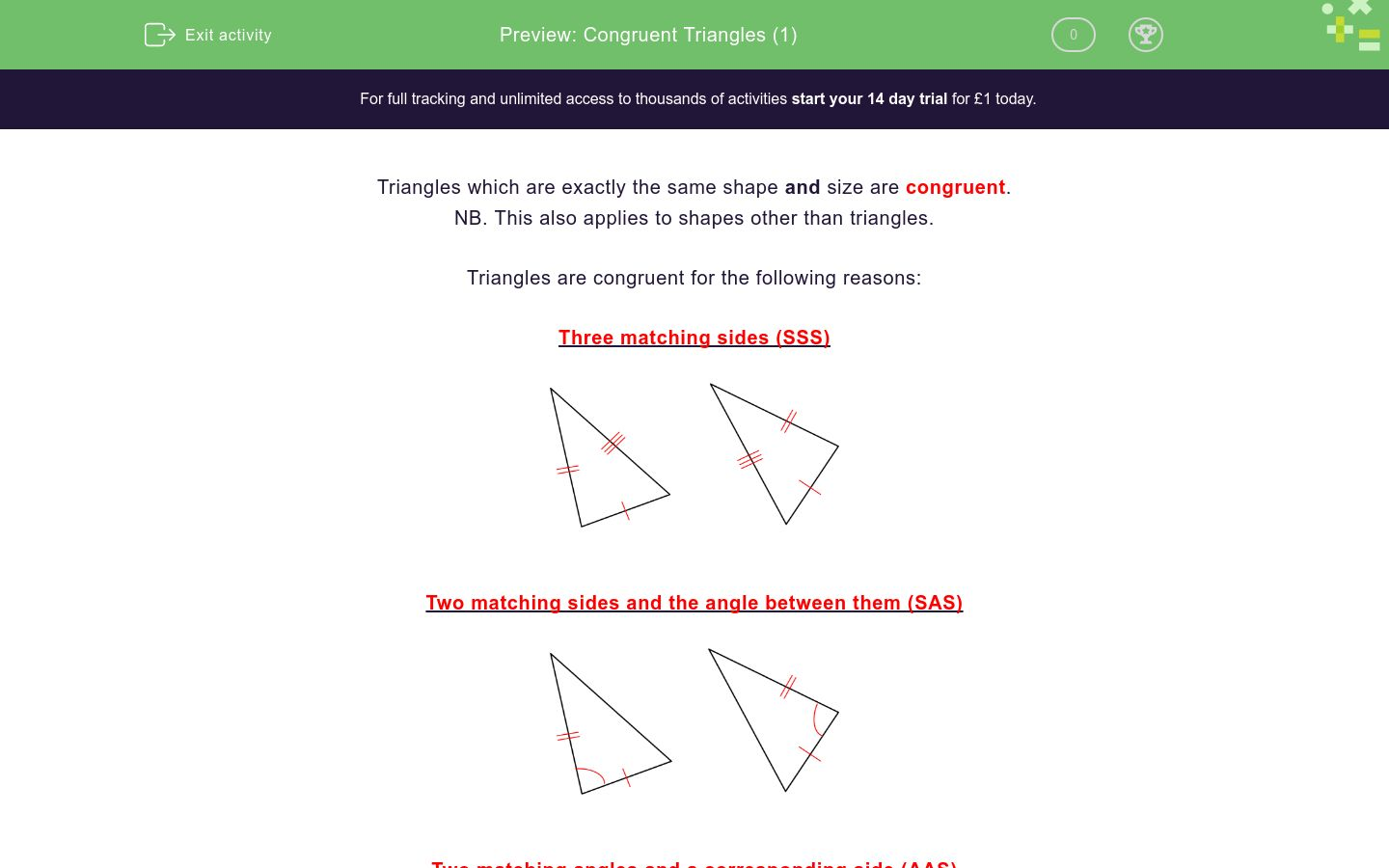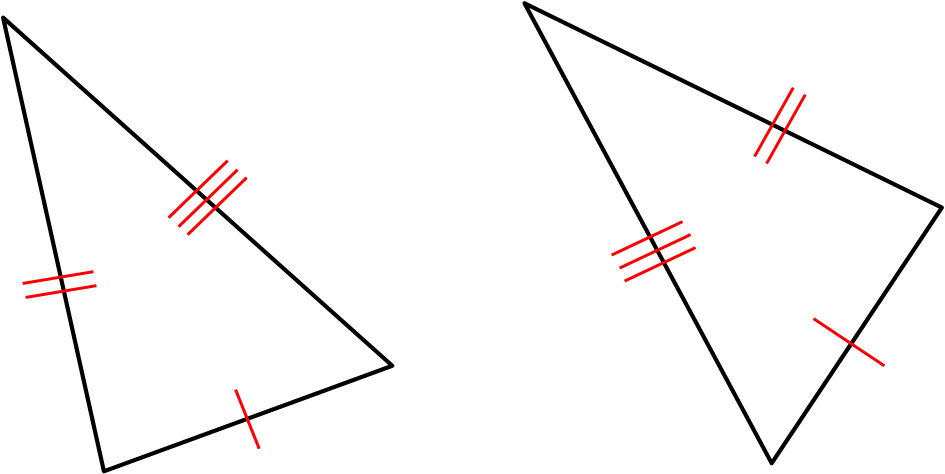# Congruent Triangles (1)

In this worksheet, students identify congruent triangles and give reasons for their congruency.Key stage:  KS 4

Curriculum topic:  Geometry and Measures

Curriculum subtopic:  Apply the Concepts of Congruence and Similarity

Difficulty level:### QUESTION 1 of 10

Triangles which are exactly the same shape and size are congruent.

NB. This also applies to shapes other than triangles.

Triangles are congruent for the following reasons:

Three matching sides (SSS)Two matching sides and the angle between them (SAS)Two matching angles and a corresponding side (AAS)In a right-angled triangle, the hypotenuse and one matching side (RHS)Look at these two triangles and select the option which matches the situation.

If the triangles are congruent, select the option with the reason for them being congruent.(not drawn to scale)

Not congruent

Congruent (SSS)

Congruent (SAS)

Congruent (AAS)

Congruent (RHS)

Look at these two triangles and select the option which matches the situation.

If the triangles are congruent, select the option with the reason for them being congruent.(not drawn to scale)

Not congruent

Congruent (SSS)

Congruent (SAS)

Congruent (AAS)

Congruent (RHS)

Look at these two triangles and select the option which matches the situation.

If the triangles are congruent, select the option with the reason for them being congruent.(not drawn to scale)

Not congruent

Congruent (SSS)

Congruent (SAS)

Congruent (AAS)

Congruent (RHS)

Look at these two triangles and select the option which matches the situation.

If the triangles are congruent, select the option with the reason for them being congruent.(not drawn to scale)

Not congruent

Congruent (SSS)

Congruent (SAS)

Congruent (AAS)

Congruent (RHS)

Look at these two triangles and select the option which matches the situation.

If the triangles are congruent, select the option with the reason for them being congruent.(not drawn to scale)

Not congruent

Congruent (SSS)

Congruent (SAS)

Congruent (AAS)

Congruent (RHS)

Look at these two triangles and select the option which matches the situation.

If the triangles are congruent, select the option with the reason for them being congruent.(not drawn to scale)

Not congruent

Congruent (SSS)

Congruent (SAS)

Congruent (AAS)

Congruent (RHS)

Look at these two triangles and select the option which matches the situation.

If the triangles are congruent, select the option with the reason for them being congruent.(not drawn to scale)

Not congruent

Congruent (SSS)

Congruent (SAS)

Congruent (AAS)

Congruent (RHS)

Look at these two triangles and select the option which matches the situation.

If the triangles are congruent, select the option with the reason for them being congruent.(not drawn to scale)

Not congruent

Congruent (SSS)

Congruent (SAS)

Congruent (AAS)

Congruent (RHS)

Look at these two triangles and select the option which matches the situation.

If the triangles are congruent, select the option with the reason for them being congruent.(not drawn to scale)

Not congruent

Congruent (SSS)

Congruent (SAS)

Congruent (AAS)

Congruent (RHS)

Look at these two triangles and select the option which matches the situation.

If the triangles are congruent, select the option with the reason for them being congruent.(not drawn to scale)

Not congruent

Congruent (SSS)

Congruent (SAS)

Congruent (AAS)

Congruent (RHS)

• Question 1

Look at these two triangles and select the option which matches the situation.

If the triangles are congruent, select the option with the reason for them being congruent.(not drawn to scale)

CORRECT ANSWER
Congruent (SSS)
EDDIE SAYS
Three matching sides
• Question 2

Look at these two triangles and select the option which matches the situation.

If the triangles are congruent, select the option with the reason for them being congruent.(not drawn to scale)

CORRECT ANSWER
Not congruent
EDDIE SAYS
Need another matching side to be congruent
• Question 3

Look at these two triangles and select the option which matches the situation.

If the triangles are congruent, select the option with the reason for them being congruent.(not drawn to scale)

CORRECT ANSWER
Not congruent
EDDIE SAYS
Not enough information matches
• Question 4

Look at these two triangles and select the option which matches the situation.

If the triangles are congruent, select the option with the reason for them being congruent.(not drawn to scale)

CORRECT ANSWER
Congruent (SAS)
EDDIE SAYS
Two sides and the angle between them match
• Question 5

Look at these two triangles and select the option which matches the situation.

If the triangles are congruent, select the option with the reason for them being congruent.(not drawn to scale)

CORRECT ANSWER
Congruent (RHS)
EDDIE SAYS
Hypotenuses and matching sides of the right-angled triangles are equal
• Question 6

Look at these two triangles and select the option which matches the situation.

If the triangles are congruent, select the option with the reason for them being congruent.(not drawn to scale)

CORRECT ANSWER
Not congruent
EDDIE SAYS
These are similar but not congruent. The sides could be any size.
• Question 7

Look at these two triangles and select the option which matches the situation.

If the triangles are congruent, select the option with the reason for them being congruent.(not drawn to scale)

CORRECT ANSWER
Congruent (SAS)
EDDIE SAYS
Two sides and the angle between them match
• Question 8

Look at these two triangles and select the option which matches the situation.

If the triangles are congruent, select the option with the reason for them being congruent.(not drawn to scale)

CORRECT ANSWER
Not congruent
EDDIE SAYS
The sides do not necessarily match
• Question 9

Look at these two triangles and select the option which matches the situation.

If the triangles are congruent, select the option with the reason for them being congruent.(not drawn to scale)

CORRECT ANSWER
Congruent (AAS)
EDDIE SAYS
Two angles and a matching side are equal
• Question 10

Look at these two triangles and select the option which matches the situation.

If the triangles are congruent, select the option with the reason for them being congruent.(not drawn to scale)

CORRECT ANSWER
Congruent (SAS)
EDDIE SAYS
Two sides and the angle between them are equal
---- OR ----

Sign up for a £1 trial so you can track and measure your child's progress on this activity.

### What is EdPlace?

We're your National Curriculum aligned online education content provider helping each child succeed in English, maths and science from year 1 to GCSE. With an EdPlace account you’ll be able to track and measure progress, helping each child achieve their best. We build confidence and attainment by personalising each child’s learning at a level that suits them.

Get started#### Similar activities you may like

Start your £1 trial today.
Subscribe from £10/month.